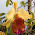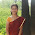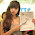## Monday, January 30, 2012

### Closest pair of points - AlgorithmCredit: wikipedia
Problem Statement : The closest pair of points problem or closest pair problem is a problem of computational geometry: given n points in metric space, find a pair of points with the smallest distance between them.

Basically you are given N points in a plane - and you ahve to find two closest points.

This is algorithm may be used to find the nearest location  given your current geo location. This question was posted to me as -

'Write the algorithm to find the nearest Star bucks coffee shop given your lat longs'

The first things that comes to the mind is a brute force approach, which is O(n^2) and may not be ideal.

```minDist = infinity
for each p in P:
for each q in P:
if p ≠ q and dist(p, q) < minDist:
minDist = dist(p, q)
closestPair = (p, q)
return closestPair

```

So, can we optimize it? Yes, lets get it in O(n log n).

This algorithm very similar to what we do in Merge Sort. The algorithm described here uses a divide and conquer approach to find out the closest  pair of points in a plane. It takes an input array of points of the type described as input  above

PSEUDO CODE

`P set of points - SORTED by X-axis`
```procedure findclosest ( P, int n)
{ n is the number of elements in set P }
Set: PLeft, PRight, Pleftmin, Prightmin, Pclosest
if (n  <= 3)
return SHORTEST(P)
else
PLeft = { p(1), p(2), …. , p(n/2) }
PRight = { p(n/2 + 1), ……. , p(n) }
Pleftmin = findclosest (PLeft , n/2)
Prightmin = findclosest (PRight , n/2)
Pclosest = MERGEPLANES (Pleftmin, Pleft, Prightmin, Pright)
return Pclosest
endif
endprocedure

procedure MERGEPLANES (P1min, P1, P2min, P2min)

D = MINIMUM among (P1min, P2min)

There might be points in each around mid-x-axis that do not form the minimum on left/right but if joined across points on other side - might give min.
We create a rectange to consider such points.

xi - xj < D == Means any 2 points whoes x-axis coordinates are less than D distance apart.
yi +D > yj > yi – D = means any 2 points with y-coordinates are less than D distance apart. Considering above and below 7-axis, means they lie in 2D range.

for (every i in P1)
for every j in P2 such that
xi - xj < D AND  (yi +D > yj > yi – D )
if DISTANCE (i, j) <  D
return (i, j)
endfor
endfor

endprocedure

```

Inside loop will at the max have 6 elements falling in that range. O(constant)
Outer loop worst case goes n times. O(n)
Total complexity of MERGEPLANES - O(n) * O(constant) = O(n)
Recursive loop - O(log n)

Thus, Complexity - O(n log n)

Some places it is also said that it may be O(n)
It is because only points in both the planes which are not more than MinDistance distance apart will have to be considered in order to find the closest pair.

Explanation

```- Say P is a set of points with x and y coordinates.
- sort all points by by x-coordinate (Write a comparatr which does that)
- Recursively, devide the list into halves. Pleft, Pright
- pass halved lists recursively to the function. We follow bottoms up approach. We'll keep deviding till we reach base case of 2-3 points and we find nearest points amont them.
- Then while keep coming out of recursion, we keep maitainining the closest pair amont Pleft and Pright.
- leftClosest = recursive(Pleft)
- rightClosest = recursive(Pright)
- Sat we have ClosestPoint data structure: - that has Point1, Point2, distance
- base case: if list.length < 3 -- return Shortest Distance ClosestPoint.
- now we have closest point from left & right halves.
- closest point will be either one of them, or points joining across the deviding line
- ClosestPoint c = merge( leftClosest, Pleft, rightClosest, Pright )
- merge
-- D = min distance from leftClosest, rightClosest
-- find min reactangle around deviding line
-- for(i: 0-Pleft.len)
--- for (j: 0-Pright.len)
----  if(Mod(pi.x-pj.x) < D || Mod(pi.y-pj.y) < d)
-----   d = Distance(...)
-----   if(d < D)
------    maintain minDistance and closest pair

```

Some places it is also said that it may be O(n)
It is because only points in both the planes which are not more than MinDistance distance apart will have to be considered in order to find the closest pair.

References

•  http:people.cis.ksu.edu/~subbu/Papers/Closest%20pair.pdf
•  http:en.wikipedia.org/wiki/Closest_pair_of_points_problem

1.Hey Ashish - Good article. I like your site.
You have a typo in your procedure MERGEPLANES (P1min, P1, P2min, P2min).
The last parameter should be P2

2.3.These provided information was really so nice,thanks for giving that post and the more skills to develop after refer that post. Your articles really impressed for me,because of all information so nice.

SAP training in Chennai

4.Great and useful article. Creating content regularly is very tough. Your points are motivated me to move on.

SEO Company in Chennai

5.“Đúng vậy, nhất định là như thế”. Cô tức giận nhìn cái bụng lộ ra của mình, đột nhiên nhìn thấy mình trông thật xấu, người toàn thị là thịt.
Đáng giận! Trước kia cô có thân hình như người mẫu vậy mà hiện tại do mang thai nên trở nên xấu xí thế này.
Nhưng tại sao c Tiếng anh cho người đi làm
Tiếng anh cấp tốc
Luyện thi toeic tại hà nội
Tiếng anh cho người lớn tuổi
Tiếng anh cho người mới bắt đầu
Học tiếng anh tại hà nội
dạy tiếng anh cho doanh nghiệpô lại để ý việc anh đi với người phụ nữ khác? Rồi lại để ý đến thân hình của mình xấu đi? Việc anh ta đi với người phụ nữ khác thì có liên quan gì đến cô? Hiện tại cô đang tranh giành tình nhân với người phụ nữ khác sao?

6.Estimate found the site and now I sit here all my free time thinking about working out. Interesting? Okay, share a link
topnotchreal casino You can not thank

7.Thank you so much for posting this. I really appreciate your work. Keep it up. Great work!Best software training company with placement in Hyderabad

8.9.Attend The Analytics Course in Bangalore From ExcelR. Practical Analytics Course in Bangalore Sessions With Assured Placement Support From Experienced Faculty. ExcelR Offers The Analytics Course in Bangalore.
ExcelR Analytics Course in Bangalore